# ASAM: Adaptive Sharpness-Aware Minimization for Scale-Invariant Learning of Deep Neural Networks

By Jungmin Kwon Samsung Research Global AI Center
By Jeongseop Kim Samsung Research Global AI Center
By Hyunseo Park Samsung Research Global AI Center
By In Kwon Choi Samsung Research Global AI Center

Recently we proposed a new optimization algorithm called Adaptive Sharpness-Aware Minimization (ASAM), which pushes the limit of deep learning via PAC-Bayesian theory. ASAM has been improving generalization performance for various tasks leveraging geometry of loss landscape, which is highly correlated with generalization. In recognition of its theoretical contribution and practicality, our paper was published at the 38th International Conference on Machine Learning (ICML 2021).

## Motivation

Generalization means how well a trained deep neural network can classify or forecast unseen data. Nowadays, deep learning has made paradigm shifts in many academic or industrial areas utilizing generalization power of deep neural networks. Thus, boosting generalization power of deep neural networks via introduction of proper training techniques has been a main goal in many studies on deep learning.

To achieve this goal, deep learning finds a (local) minimum for a loss surface of training data by using gradient-based optimizers e.g. stochastic gradient descent (SGD) or Adam, which indirectly induces minimization of generalization loss.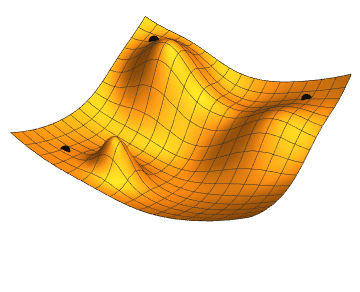Figure 1 Gradient descent with 3 different initial conditions.

To guarantee this indirect minimization of generalization loss, however, sufficiently large training data and large model size are required. In many practical cases, sufficiently large data and model size are not available, which limits the generalization power of deep neural networks.

To go one step further beyond only minimizing the training loss, here we design an optimizer which enhances the generalization ability of trained neural networks. This improvement of generalization power can contribute to data efficiency and reduction of required model size.

## Background

A description of the correlation between the generalization gap and the sharpness of loss function is a good starting point for the discussion of the ASAM algorithm. The generalization gap and sharpness of training loss can be defined as follows: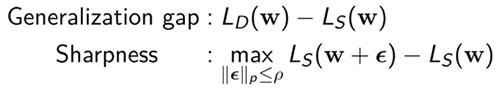where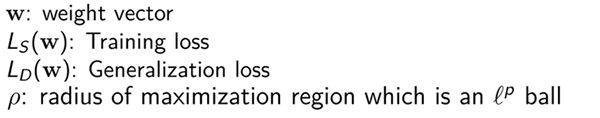As can be seen in the definition above, the generalization gap is defined as the difference between the generalization loss and the training loss. Thus, a large generalization gap means that a neural network has weak generalization power. The sharpness of loss function can be defined as the difference between the maximum training loss in an ${\ell}^p$ ball with a fixed radius $\mathbf{ρ}.$ and the training loss at $\mathbf{w}.$

The paper  shows the tendency that a sharp minimum has a larger generalization gap than a flat minimum does. The following figure is a conceptual sketch showing the positive correlation between the sharpness and generalization gap.Figure 2 A conceptual sketch of sharp and flat minima .

This tendency has also been investigated and verified via works based on PAC-Bayesian theory in the literature (, ). Inspired by the sharpness and the generalization gap,  suggests sharpness-aware minimization (SAM). SAM aims to minimize the local maximum of the training loss in epsilon-ball of $\parallel{ϵ}\parallel_2 ≤ ρ$, which can be decomposed into the sharpness and training loss, by solving the following minimax problem to minimize the generalization loss indirectly.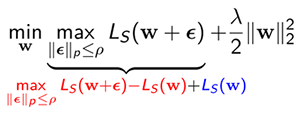However,  points out that this kind of sharpness with a fixed radius could have a weak correlation with the generalization gap due to vulnerability to weight scaling. This issue becomes more pronounced when a rectifier activation function such as ReLU is used. As in the following example, if the weight parameters before and after a ReLU activation function is multiplied by a scaling factor a and $\frac{1}{a}$, respectively, the generalization power is not affected by the scaling factor, whereas the sharpness changes with the scaling factor.

EX) $f\left(x;a\right)=\frac{1}{a}w_1ReLU(aw_2x)$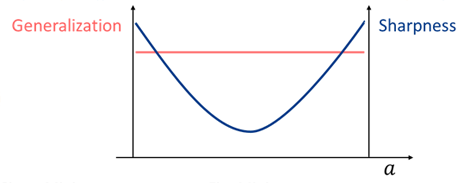Figure 3 Change of generalization power and sharpness with respect to scaling factor $a$

This vulnerability to weight scaling of sharpness is the main cause that sharpness has a weak correlation with generalization, which prevents SAM from guaranteeing sufficiently strong generalization power of neural networks.

## Adaptive Sharpness: A Scale-Invariant Generalization Measure

To strengthen the correlation by eliminating the vulnerability to weight scaling, we introduce the concept of adaptive sharpness defined as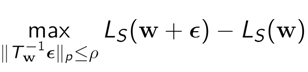where ${T}_\mathbf{w}^{-\mathbf{1}}$ is a normalization operator which can cancel out the weight scaling effect. Since the maximization region $\parallel{T}_\mathbf{w}^{-\mathbf{1}}ϵ\parallel ≤ ρ$ is adjusted with respect to positions in weight space, in contrast to the sharpness, the adaptive sharpness can be independent of weight scaling like generalization power as can be seen in Table 1. Through correlation analysis including granulated coefficients ψ and Kendall rank coefficients τ which are correlation measures suggested by  as well as scatter plots, we can demonstrate that the adaptive sharpness has stronger correlation compared to the sharpness.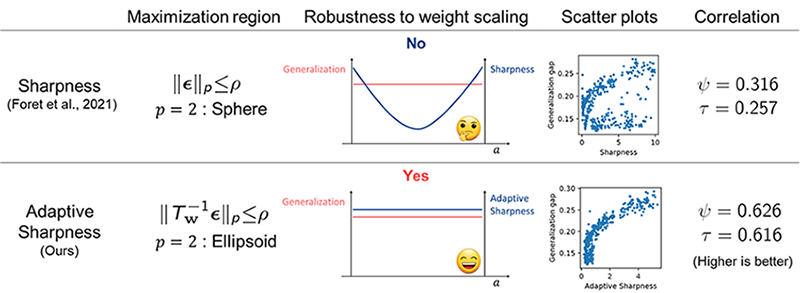Table 1 Comparison between sharpness and adaptive sharpness.

Using the concept of adaptive sharpness, we can formulate the Adaptive Sharpness-Aware Minimization (ASAM) problem as follows.By minimizing the local maximum of the training loss in $\parallel{T}_\mathbf{w}^{-\mathbf{1}}ϵ\parallel_2 ≤ ρ$, which can be decomposed into the adaptive sharpness and training loss, ASAM induces the minimization of generalization loss without the vulnerability to weight scaling. Also due to the negligible calculation cost of $T_\mathbf{w}^{-1}$, ASAM can be considered as efficient as SAM.

The following figure shows behaviors of SAM and ASAM on loss contours of a simple neural network.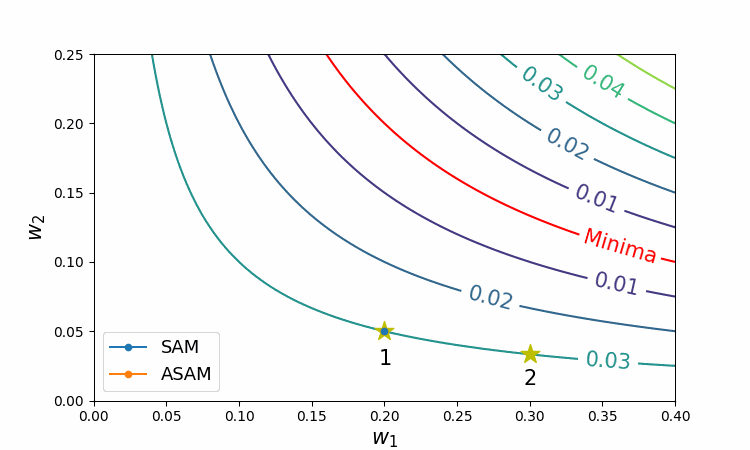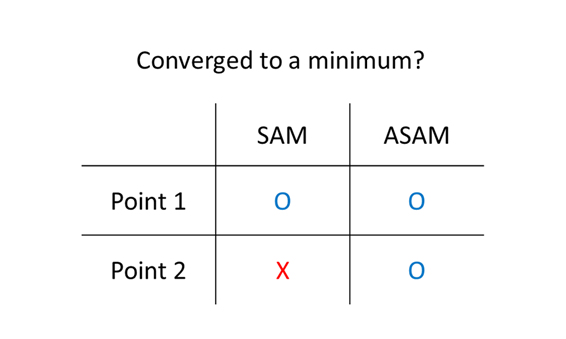Figure 4 Trajectories of SAM and ASAM

As can be seen in this figure, ASAM does not depend on the initial weight by benefiting from adjusting the region, whereas SAM may fail to converge to the valley entirely.

## Experimental Results

We also empirically show that ASAM consistently outperforms SGD and SAM in the tests on CIFAR  and ImageNet  datasets using various neural network architectures. Moreover, for CIFAR-100 dataset, Pyramidnet-272  trained with ASAM shows the state-of-the-art performance among the models using no extra data.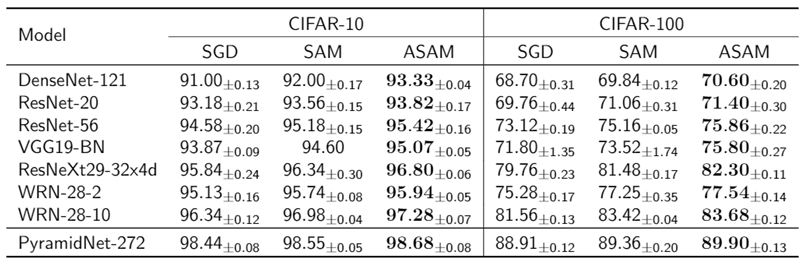Table 2 Maximum test accuracies on CIFAR-{10, 100}.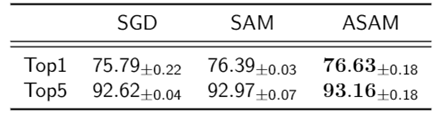Table 3 ImageNet using ResNet-50.

In the test of IWSLT2014  which is a dataset of machine translation task, we could observe that generalization performance of transformer  can be improved by ASAM combined with Adam optimizer .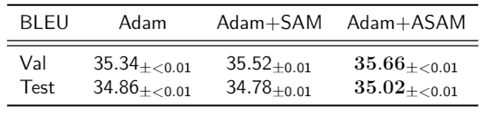Table 4 IWSLT’14 DE-EN using Transformer.

These results imply that ASAM can enhance generalization performance of deep neural network architectures in various tasks beyond the image classification.

## Usability of ASAM

Another strength of ASAM is that it is an easy-to-apply, general-purpose optimizer like SGD or Adam. The code on the left shows a PyTorch example of typical training codes using SGD and we can improve the performance just by adding 4 lines to it as can be seen in the right code.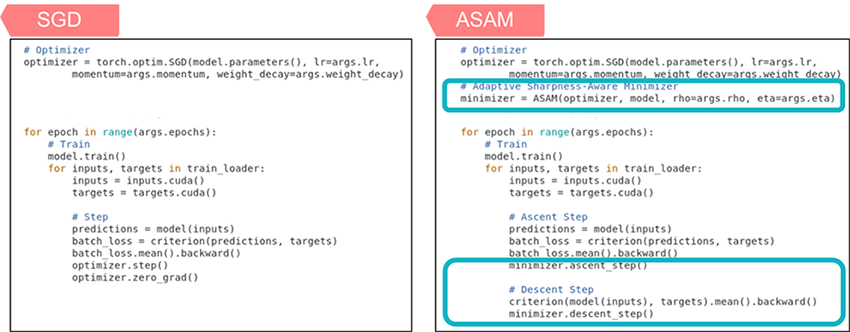Figure 5 PyTorch examples of training codes: SGD and ASAM.

## Conclusions

In the field of deep learning, optimization techniques (SGD, Adam, etc.) are one of the key factors influencing the performance of deep neural network models. As shown in this study, the development of such optimization techniques can generally improve the performance of models for various tasks, and the performance can be improved just by replacing the optimization technique without modifying the model structure, so we expect significant positive impact from ASAM. Contributing to the development of core technologies in the field of deep learning, ASAM will serve as a stepping stone to raise the level of quality of our products equipped with various AI models.

## Publication

https://arxiv.org/abs/2102.11600

Github URL

https://github.com/SamsungLabs/ASAM

## Reference

 Keskar, N. S., Nocedal, J., Tang, P. T. P., Mudigere, D., and Smelyanskiy, M. On large-batch training for deep learning: Generalization gap and sharp minima. In 5th International Conference on Learning Representations, ICLR 2017, 2017

 Dziugaite, G. K., & Roy, D. M. (2017). Computing nonvacuous generalization bounds for deep (stochastic) neural networks with many more parameters than training data. arXiv preprint arXiv:1703.11008.

 Jiang, Y., Neyshabur, B., Mobahi, H., Krishnan, D., & Bengio, S. (2019, September). Fantastic Generalization Measures and Where to Find Them. In International Conference on Learning Representations.

 Foret, P., Kleiner, A., Mobahi, H., & Neyshabur, B. (2020, September). Sharpness-aware Minimization for Efficiently Improving Generalization. In International Conference on Learning Representations.

 Dinh, L., Pascanu, R., Bengio, S., & Bengio, Y. (2017, July). Sharp minima can generalize for deep nets. In International Conference on Machine Learning (pp. 1019-1028). PMLR.

 Krizhevsky, A., Nair, V., and Hinton, G. (2009). CIFAR-10 and CIFAR-100 datasets. URL: https://www.cs.toronto.edu/~kriz/cifar.html.

 Deng, J., Dong, W., Socher, R., Li, L.-J., Li, K., and Fei-Fei, L. (2009). ImageNet: A large-scale hierarchical image database. In 2009 IEEE conference on computer vision and pattern recognition, pp. 248–255. IEEE.

 Han, D., Kim, J., & Kim, J. (2017). Deep pyramidal residual networks. In Proceedings of the IEEE conference on computer vision and pattern recognition (pp. 5927-5935).

 Cettolo, M., Niehues, J., Stüker, S., Bentivogli, L., and Federico, M. (2014). Report on the 11th IWSLT evaluation campaign, IWSLT 2014. In Proceedings of the International Work-shop on Spoken Language Translation, Hanoi, Vietnam, volume 57.

 Vaswani, A., Shazeer, N., Parmar, N., Uszkoreit, J., Jones, L., Gomez, A. N., Kaiser, L., and Polosukhin, I. (2017). Attention is all you need. In NIPS.

 Kingma, Diederik P., and Jimmy Ba. (2014). Adam: A method for stochastic optimization. arXiv preprint arXiv:1412.6980.